# Solving Nonlinear Equations Iteratively

Problem

You'd like to solve a nonlinear equation that requires an iterative solution and are not sure how to approach it in Excel.

Solution

Use Goal Seek or Solver. See the introduction to this chapter for more information on how these two tools differ as well as their respective advantages and disadvantages.

Discussion

In this recipe, I want to show you by way of example how to use Excel's built-in Solver and Goal Seek tools to solve a nonlinear equation. The equation we'll consider is: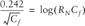This equation is used to estimate a frictional drag coefficient, Cf, as a function of the Reynolds number , RN, for some ship resistance calculations. There are other equations for this same purpose but I chose this classical one because it can't be written in an explicit form of y = g(x). Instead, you have to resort to some iterative method to find a value of Cf corresponding to some given value of RN.

I'll show you how to use both Goal Seek and Solver to solve this example problem. In both cases the equation must first be rearranged in the following form: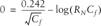Now we can use Solver or Goal Seek to iteratively find a value for Cf given a value for RN that makes the righthand side of this equation equal to 0. But first, you need to enter the righthand side of this equation as a spreadsheet formula, as shown in Figure 9-7.

Figure 9-7. Nonlinear equation example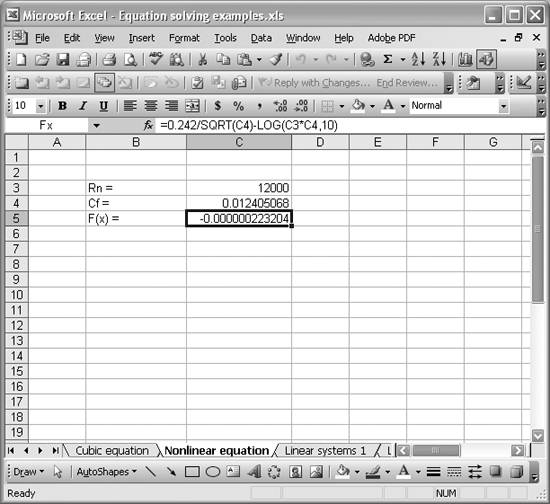Cell C5 contains a worksheet formula representing the righthand side of our equation. The formula is =0.242/SQRT(C4)-LOG(C3*C4,10), as shown in the formula bar in Figure 9-7. Cell C3 contains the given Reynolds number, while cell C4 contains the corresponding friction coefficient, found using either Solver or Goal Seek. Before actually using Solver or Goal Seek, you should enter your initial guess for the friction coefficient in cell C4. As mentioned in the introduction to this chapter, you should pick an initial guess that is reasonable for the problem at hand.

Finding Cf with Goal Seek

To use Goal Seek to find a solution for this example, select Tools [Image_Link]/images/U2192.jpg border=0> Goal Seek...from the main menu bar to open the Goal Seek window shown in Figure 9-8.

Figure 9-8. Goal Seek window for nonlinear equation example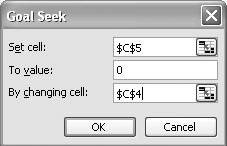In the "Set cell" field, type in C5, or press the little icon to the right of the edit field to temporarily go back to the spreadsheet and click cell C5 to select it (press Enter when you've selected it to return to the Goal Seek window). Remember, cell C5 contains the formula representing the righthand side of our equation, and we want the result of this formula to be zero. Therefore, in the "To value" field, type in 0. That's our target value. Now, set the "By changing cell" field to C4, which contains the friction coefficient value. When you press the OK button, Goal Seek will vary the value in C4 until the formula in C5 is approximately 0. The result for this example is shown in Figure 9-7, where the residual shown in cell C5 is -2.23 x 10-7.

I should mention that my initial guess (i.e., the value I started with in cell C4) was 1 x 10-7. I would have chosen 0 since I know the friction coefficient should be a pretty small number, but I didn't, because it would have resulted in a divide-by-zero error for this problem. Running Goal Seek with the divide-by-zero error would result in Goal Seek failing with a "Formula in cell must result in a number" error.

A residual of -2.23 x 10-7 isn't that bad, but we can do a little better. As discussed in the introduction, you can change the convergence settings for Goal Seek by going to the Options window. Select Tools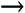Options...from the main menu bar to open the Options window (shown earlier in Figure 9-2). On the Calculation panel, I checked Iteration, set "Maximum iterations to 5,000, and set "Maximum change" to 1 x 10-8. With these settings, Goal Seek finds a solution with a much smaller residual, 1.2 x 10-13. The corresponding coefficient of friction for the given Reynolds number is 0.0124.

Finding Cf with Solver

Goal Seek works well for this simple example, and in practice you would not have to rely on Solver for this problem. For illustration purposes, however, I want to show you how to apply Solver to the same problem. The spreadsheet set up in this case is the same as in the case of using Goal Seek. All you do differently is select Tools [Image_Link]/images/U2192.jpg border=0> Solver...from the main menu bar to open Solver instead of Goal Seek. The Solver Parameters window for this example is shown in Figure 9-9.

Figure 9-9. Solver Parameters window for nonlinear equation example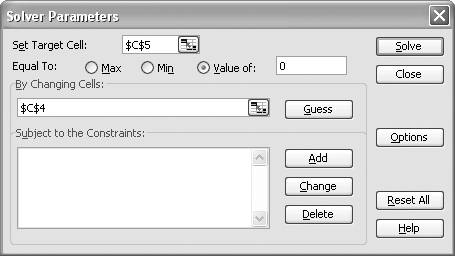Here, I set the Set Target Cell field to C5 and set the Equal To option to a value of 0. Again, C5 contains the righthand side of our equation, which we want equal to 0. Next, I set the By Changing Cells field to cell C4, which contains the initial value for the friction coefficient. Pressing the Solve button initiates the solution process.

Solver does indeed find a solution, yielding a friction coefficient of 0.0124 with a residual of -4.87 x 10-12. These values are comparable with the results obtained using Goal Seek.

Just for fun, try this example again, but this time use an initial guess for the friction coefficient of 1 or 5 or some large number. In this case, Solver actually fails to find a solution! What happens is that Solver overshoots the actual solution and ends up trying a value for the friction coefficient of 0 for an iteration. This of course yields a divide by zero error, which causes Solver to fail prematurely. This little exercise highlights the importance of selecting a reasonable initial guess. It further shows that if your first attempt fails, you should try a different initial guess as the new one might work.Excel Scientific and Engineering Cookbook (Cookbooks (OReilly))
ISBN: 0596008791
EAN: 2147483647
Year: N/A
Pages: 206
Authors: David M Bourg

Similar book on Amazon